# Algebra 1 : How to find out if lines are parallel

## Example Questions

← Previous 1 3 4 5 6 7 8 9 10 11

### Example Question #1 : How To Find Out If Lines Are Parallel

Which of the following lines are parallel to y = 2x + 5?

y = 4x + 3

y = (–1/2)x + 6

y = 2x – 3

y = 6x + 5

y = –2x + 8

y = 2x – 3

Explanation:

Lines are parallel if they have the same slope. The lines are all given in the form y = mx + b. In this form, m represents the slope, thus, we are looking for another line with a slope of 2. This is y = 2x – 3.

Note that the line given by y = (–1/2)x + 6 has a slope that is the negative reciprocal of 2. This line will be perpendicular to our given line.

### Example Question #2 : How To Find Out If Lines Are Parallel

True or False.

The following two lines are parallel.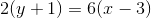True

False

True

Explanation:

When both equations are solved for the formthe slopes are the same.

### Example Question #3 : How To Find Out If Lines Are Parallel

Which of the following lines are parallel to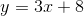?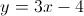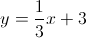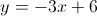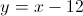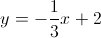Explanation:

Parallel lines must have identical slopes. The given equation is in theform. Therefore, we can quickly determine that its slope is 3. The only other equation with a slope of 3 is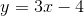.

### Example Question #1 : How To Find Out If Lines Are Parallel

Which of these lines is parallel to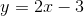?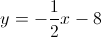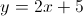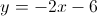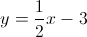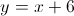Explanation:

Parallel lines have the same slope. Since all of these equations are in theform, it is easy to determine their slopes,. The slope ofis 2.

The only other line with a slope of 2 is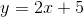.

### Example Question #1 : How To Find Out If Lines Are Parallel

Which of these lines is parallel to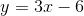?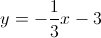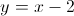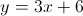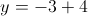Explanation:

Parallel lines must have equal slopes to one another. Since all of the lines are in theform, it is easy to determine their slopes. The given line has a slope of 3, which means that any line that is parallel to it must have a slope of 3. The only other line with a slope of 3 is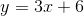.

### Example Question #6 : How To Find Out If Lines Are Parallel

Which of these pairs of lines are parallel?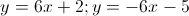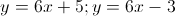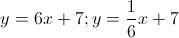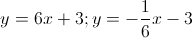Explanation:

Parallel lines have the same slope as one another. The only pair of lines with the same slope is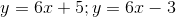.

### Example Question #2 : How To Find Out If Lines Are Parallel

Which of these lines is parallel to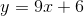?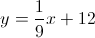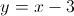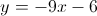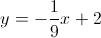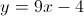Explanation:

Parallel lines have identical slopes with one another. The given line has a slope of 9, so its parallel line must also have a slope of 9. The only other line with a slope of 9 is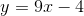.

### Example Question #8 : How To Find Out If Lines Are Parallel

Which of these lines is parallel to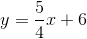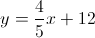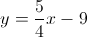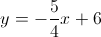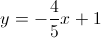Explanation:

Parallel lines have identical slopes. Since all of these lines are in theform, you can easily determine their slopes (). The slope of the given line is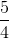, so a line that's parallel to it must have the same slope. The only other line with a slope ofis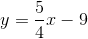.

### Example Question #9 : How To Find Out If Lines Are Parallel

Which of these lines is parallel to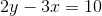?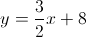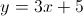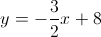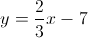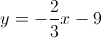Explanation:

Parallel lines have identical slopes, so you must figure out which of these lines have the same slope as the given line. To determine the slope of the given line, you can rearrange it to resemble theform.can be rearranged to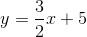. Looking at the rearranged equation, it is clear that the line's slope is. The only other line with a slope ofis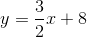.

### Example Question #10 : How To Find Out If Lines Are Parallel

Two lines are parallel to each other. One of the lines has an equation of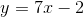. What could be the equation of the other line?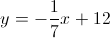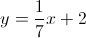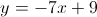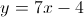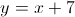Parallel lines have identical slopes to one another. The slope of the given line is 7, so the slope of the other line must also be 7. The only other equation with a slope of 7 is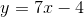.